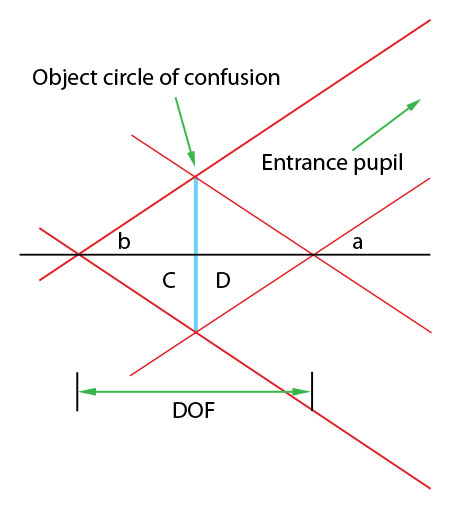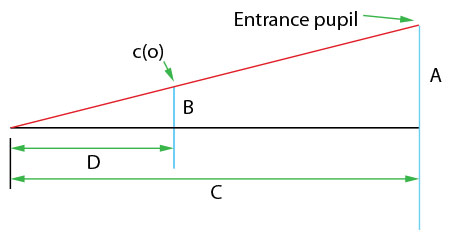# Derivation:Macro DOF equation

back

I was recently looking at a depth of field diagram like the one in the last post and decided that I wanted to figure out the macro DOF equation in my own way. I know that the typical macro DOF equation is a simplification and approximation of the real DOF equation that is much more complicated. So I went about looking at the diagram to see how it can be simplified.

The simplification that I saw was that with a sufficiently small DOF, the angle that the edge of the exit pupil makes with an object at the front of the DOF is very nearly the same as it makes with an object at the back of the DOF (diagram below: angle a is very nearly the same as angle b). Since these angles are very close to the same, I can make the assumption that they are the same. That means that the triangles made by the rays going through the edge of the object circle of confusion, c(o), from the front and the back are the same (diagram: triangle C is very nearly the same as triangle D). This assumption means that I can just worry about the amount of DOF on the back side of the focus plane and multiply it b 2.This leads to a simplification where you can calculate the DOF using similar triangles:DOF = 2*D

Given the similar triangles:

A/B = C/D

D = C*B/A

DOF = 2*C*B/A

A = En/2 = f/2N (where En = entrance pupil, f = focal length, N = aperture setting)

B = c(o)/2 = c/2*m (where c(o) = object circle of confusion, c = circle of confusion, m = mangification).

C is very nearly equal to the focus distance since the DOF is quite small and this equation only applies to higher magnifications.

C = f*((1/m)+(1/P)) (where f = focal length, m = magnification, P = pupillary magnification)

All these can be put into the original equation an you get:

DOF = 2 * (c/2m) * f *(1/m + 1/P)/(f/2*N)

DOF = 2*c*N*f*(1/m + 1/P)/m*f

DOF = 2*c*N*(1/P + 1/m)/m (moving things around, f's and a couple 2's cancel out)

DOF = 2*c*N*m*(1/P + 1/m)/m^2 (multiply everything by m/m)

DOF = 2*c*N*(m/P + 1)/m^2 (move the m into the 1/P + 1/m part)

This is not quite like the typical DOF at macro equation in that it includes P, although it will be more accurate if you include P. Most of the time P is considered to be 1 and the equation turns into the one typically seen.

DOF = 2*c*N*(m+1)/m^2